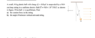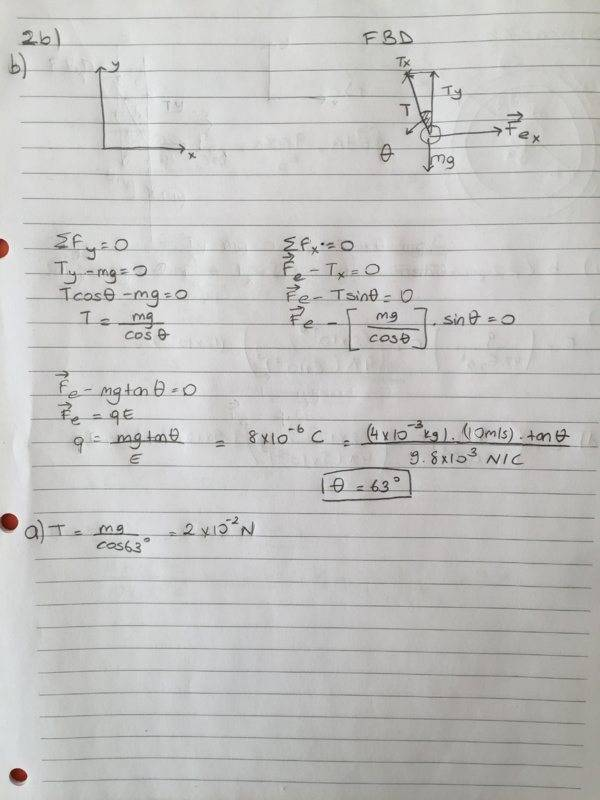# Electric Field's Force on a Suspended Plastic Ball

EsinDerin
Homework Statement:
A small, 4.0-g plastic ball with charge Q=8uC,is suspended by a
cm-long string in a uniform electric field ⃗ E=9.8x10^2 in figure. If the ball is in equilibrium, Find
a) the tension force in the string
b) the angle between vertical axis and string.
Relevant Equations:
E=k*q/r^2
k=1/4Eopi
Hi,I couldn't do this problem.I hope someone could help meths is my first time in this forum

Homework Helper
Gold Member
2022 Award
You need to show us how much you can do. You must have some idea how to solve this?

Mentor
Welcome to the PF.Homework Statement:: A small, 4.0-g plastic ball with charge Q=8uC,is suspended by a
cm-long string in a uniform electric field ⃗ E=9.8x10^2 in figure. If the ball is in equilibrium, Find
a) the tension force in the string
b) the angle between vertical axis and string.
Relevant Equations:: E=k*q/r^2
k=1/4Eopi

Hi,I couldn't do this problem.I hope someone could help meths is my first time in this forum
In addition to showing your work, please try again to attach the diagram of the problem. How many cm long is the string?

EsinDerin
This is the question

#### Attachments

•Ekran Resmi 2020-04-06 23.50.56.png
16 KB · Views: 108
Mentor
Ah, that helps. Okay, please show us your free body diagram (FBD) for the plastic ball, and write the equation for the force of the electric field on a charged body (it is similar to the equation you wrote for the electric field from a charged particle above). Please show us your work. Thanks.

EsinDerinI'm not sure about the solution.If there is any mistake,please show it.Thanks :)

Homework Helper
Gold Member
2022 Award
View attachment 260181
I'm not sure about the solution.If there is any mistake,please show it.Thanks :)
It all looked good until you came to the calculation. Why didn't you do:
$$\tan \theta = \frac{qE}{mg}$$
You made a mistake writing down the charge, which is ##0.8 \mu C##.

One minor point is that you shouldn't mix vectors like ##\vec{F_e}## and scalars like ##T_x## in the same equation.

•berkeman
EsinDerin